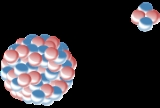xAlpha decayOverview

Alpha decay is a type of radioactive decay in which an atomic nucleus
Atomic nucleus
The nucleus is the very dense region consisting of protons and neutrons at the center of an atom. It was discovered in 1911, as a result of Ernest Rutherford's interpretation of the famous 1909 Rutherford experiment performed by Hans Geiger and Ernest Marsden, under the direction of Rutherford. The...

emits an alpha particle
Alpha particle
Alpha particles consist of two protons and two neutrons bound together into a particle identical to a helium nucleus, which is classically produced in the process of alpha decay, but may be produced also in other ways and given the same name...

and thereby transforms (or 'decays') into an atom with a mass number
Mass number
The mass number , also called atomic mass number or nucleon number, is the total number of protons and neutrons in an atomic nucleus. Because protons and neutrons both are baryons, the mass number A is identical with the baryon number B as of the nucleus as of the whole atom or ion...

4 less and atomic number
Atomic number
In chemistry and physics, the atomic number is the number of protons found in the nucleus of an atom and therefore identical to the charge number of the nucleus. It is conventionally represented by the symbol Z. The atomic number uniquely identifies a chemical element...

2 less. For example:

→ +

which can also be written as:

→ + .

An alpha particle
Alpha particle
Alpha particles consist of two protons and two neutrons bound together into a particle identical to a helium nucleus, which is classically produced in the process of alpha decay, but may be produced also in other ways and given the same name...

is the same as a helium-4
Helium-4
Helium-4 is a non-radioactive isotope of helium. It is by far the most abundant of the two naturally occurring isotopes of helium, making up about 99.99986% of the helium on earth. Its nucleus is the same as an alpha particle, consisting of two protons and two neutrons. Alpha decay of heavy...

nucleus
Atomic nucleus
The nucleus is the very dense region consisting of protons and neutrons at the center of an atom. It was discovered in 1911, as a result of Ernest Rutherford's interpretation of the famous 1909 Rutherford experiment performed by Hans Geiger and Ernest Marsden, under the direction of Rutherford. The...

, which has mass number
Mass number
The mass number , also called atomic mass number or nucleon number, is the total number of protons and neutrons in an atomic nucleus. Because protons and neutrons both are baryons, the mass number A is identical with the baryon number B as of the nucleus as of the whole atom or ion...

4 and atomic number
Atomic number
In chemistry and physics, the atomic number is the number of protons found in the nucleus of an atom and therefore identical to the charge number of the nucleus. It is conventionally represented by the symbol Z. The atomic number uniquely identifies a chemical element...

2.

Alpha decay is by far the most common form of cluster decay
Cluster decay
Cluster decay is a type of nuclear decay in which a parent atomic nucleus with A nucleons and Z protons emits a cluster of Ne neutrons and Ze protons heavier than an alpha particle but lighter than a typical binary fission fragment Cluster decay (also named heavy particle radioactivity or heavy...

where the parent atom
Atom
The atom is a basic unit of matter that consists of a dense central nucleus surrounded by a cloud of negatively charged electrons. The atomic nucleus contains a mix of positively charged protons and electrically neutral neutrons...

ejects a defined daughter
Decay product
In nuclear physics, a decay product is the remaining nuclide left over from radioactive decay. Radioactive decay often involves a sequence of steps...

collection of nucleon
Nucleon
In physics, a nucleon is a collective name for two particles: the neutron and the proton. These are the two constituents of the atomic nucleus. Until the 1960s, the nucleons were thought to be elementary particles...

s, leaving another defined product behind (in nuclear fission
Nuclear fission
In nuclear physics and nuclear chemistry, nuclear fission is a nuclear reaction in which the nucleus of an atom splits into smaller parts , often producing free neutrons and photons , and releasing a tremendous amount of energy...

, a number of different pairs of daughters of approximately equal size are formed).